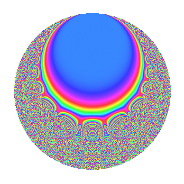# Properties

 Label 12.16.bLevel 12 Weight 16 Character orbit b Rep. character $$\chi_{12}(11,\cdot)$$ Character field $$\Q$$ Dimension 28 Newform subspaces 1 Sturm bound 32 Trace bound 0

# Related objects

## Defining parameters

 Level: $$N$$ = $$12 = 2^{2} \cdot 3$$ Weight: $$k$$ = $$16$$ Character orbit: $$[\chi]$$ = 12.b (of order $$2$$ and degree $$1$$) Character conductor: $$\operatorname{cond}(\chi)$$ = $$12$$ Character field: $$\Q$$ Newform subspaces: $$1$$ Sturm bound: $$32$$ Trace bound: $$0$$

## Dimensions

The following table gives the dimensions of various subspaces of $$M_{16}(12, [\chi])$$.

Total New Old
Modular forms 32 32 0
Cusp forms 28 28 0
Eisenstein series 4 4 0

## Trace form

 $$28q + 26968q^{4} + 823656q^{6} - 3812052q^{9} + O(q^{10})$$ $$28q + 26968q^{4} + 823656q^{6} - 3812052q^{9} - 43831600q^{10} - 226414248q^{12} + 124527272q^{13} - 1459325408q^{16} - 4234777584q^{18} - 7261350648q^{21} - 2533670160q^{22} - 18487781856q^{24} - 146804950740q^{25} + 5481093840q^{28} + 8237058960q^{30} + 204574669728q^{33} + 450165745472q^{34} + 232631927160q^{36} + 386069193224q^{37} + 1945471012160q^{40} + 1938106219632q^{42} - 5007113912640q^{45} + 2270790222432q^{46} + 5846474725152q^{48} - 18480860963084q^{49} - 6113229405424q^{52} + 1626598700568q^{54} + 8085872464056q^{57} - 19395437098192q^{58} - 10924219377600q^{60} - 16392792556696q^{61} + 21633892829056q^{64} + 4928819126448q^{66} + 137029869973056q^{69} + 91772543171040q^{70} + 66924493142592q^{72} + 158451626683736q^{73} - 205265768291280q^{76} + 58364283489648q^{78} + 116126816635836q^{81} - 750029796726880q^{82} - 92605433207856q^{84} - 30099357052160q^{85} - 619691835246912q^{88} - 471443548913520q^{90} - 777661615138584q^{93} + 1301107064491200q^{94} + 525428335287168q^{96} - 824166397720648q^{97} + O(q^{100})$$

## Decomposition of $$S_{16}^{\mathrm{new}}(12, [\chi])$$ into newform subspaces

Label Dim. $$A$$ Field CM Traces $q$-expansion
$$a_2$$ $$a_3$$ $$a_5$$ $$a_7$$
12.16.b.a $$28$$ $$17.123$$ None $$0$$ $$0$$ $$0$$ $$0$$